# Length, surface and volume

Subjects:

• Length
• Surface
• Content

Height:
Length is a one-dimensional measure. We can measure the length of all kinds of objects. We express the size of this object in a unit of length. The unit of length is also known as the measure of length. The ruler in the picture below measures a length of 5 cm.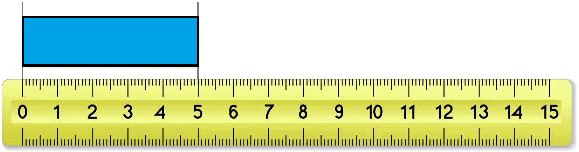The length measure can be expressed in, among others, the following units:

• millimeters (mm)
• centimeters (cm)
• decimeters (dm)
• meters (m)
• decameter (dam)
• hectometre (hm)
• kilometers (km)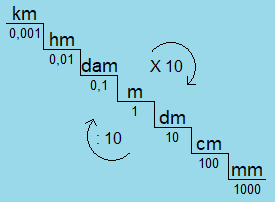Between each unit of length the step is a factor of 10. From largest to smallest we have to multiply by 10 and vice versa divide by 10.
In the image above it can be seen that 1 meter equals 0,001 km and 1000 mm.

From smallest to largest is times 10.
mm -> cm -> dm -> m -> dam -> hm -> km

From largest to smallest is dividing by 10.
km -> hm -> dam -> m -> dm -> cm -> mm

Surface:
Area is a two-dimensional measure. We express the area of ​​a given area or object in a unit of area. The area is calculated by multiplying the length by the width.
An object with a length of 8 by 5 centimeters has an area of ​​(8*5) = 40 cm².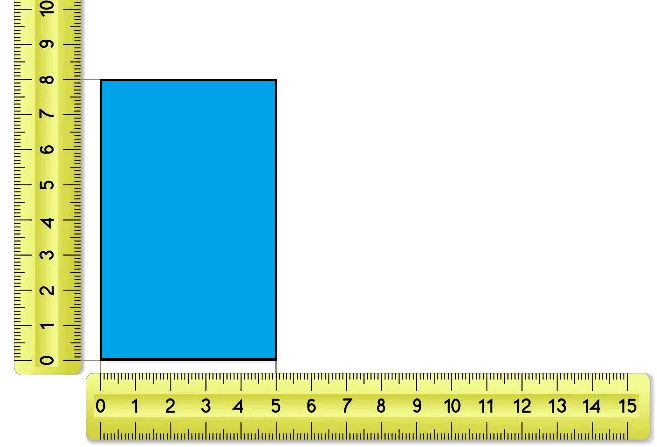We express the area in, among others, the following units:
• square millimeters (mm²)
• square centimeters (cm²)
• square decimeter (dm²)
• square meters (m²)
• square decameter (dm²)
• square hectometers (hm²)
• square kilometers (km²)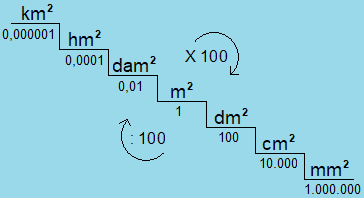In automotive technology, for example, we are dealing with the frontal area of ​​a passenger car that is 1,9 m². This is equal to 19000 cm².

From smallest to largest is times 100.
mm² -> cm² -> dm² -> m² -> dam² -> hm² -> km²

From largest to smallest is dividing by 100.
km² -> hm² -> dam² -> m² -> dm² -> cm²-> mm²

1 hm² = 1 ha.
A square hectometer (hm²) is also referred to as a hectare (ha). One hectare is an area of ​​100 x 100 meters and is equal to 10.000 m².

1 dam² = 1 are.
A square decameter (dam²) is also called an are. One are equals an area of ​​100 m².

Content:
Content is a three-dimensional measure. With regard to the content, we are dealing with the length, width and depth. Multiplying the length, width and depth gives the content. A cube with a length of 7 cm, a width of 8 cm and a depth of 8 cm has a volume of: 7*8*8 (l*w*h) = 448 cm³.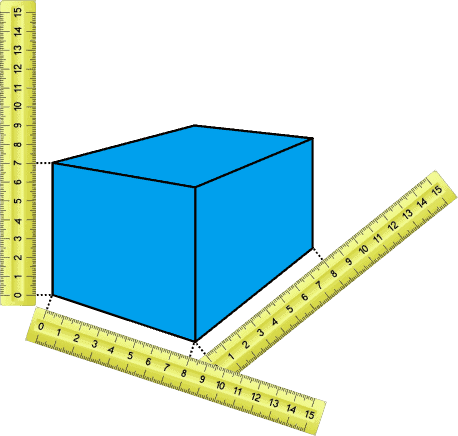We express the content in, among others, the following units:
• cubic millimeters (mm³)
• cubic centimeters (cm³)
• cubic decimeter (dm³)
• cubic meter (m³)
• cubic decameter (dm³)
• cubic hectometers (hm³)
• cubic kilometer (km³)From smallest to largest is times 1000.
mm³ -> cm³ -> dm³ -> m³ -> dam³ -> hm³ -> km³

From largest to smallest is dividing by 1000.
km³ -> hm³ -> dam³ -> m³ -> dm³ -> cm³-> mm³

1 cm³ = ml = cc.
In car technology, for example, we are dealing with the cylinder capacity of an engine that is 1988 cm³. This is equivalent to 1988 ml and 1988 cc.

cm³ to dm³ = liters.
Looking at the table, the multiplication factor between cm³ and dm³ is 1000. If we convert cm³ to dm³, we get 1,988 dm³ which is equal to 1,988 liters.

error: Alert: Content is protected !!